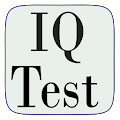# IQ and Aptitude Test Practice APK

## org.aztest.iqtest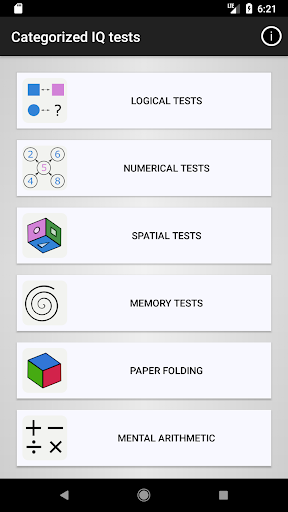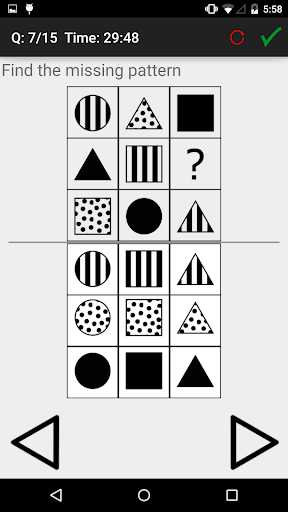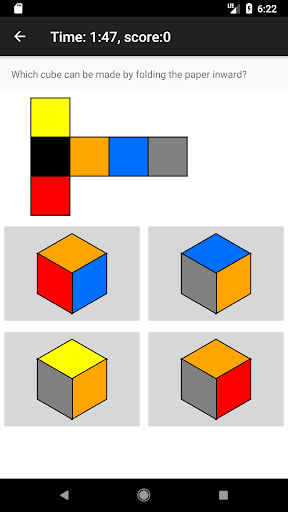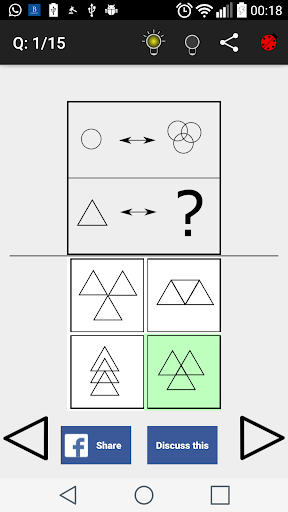Call it an Intelligence test, IQ test, aptitude test, or psychometric test, they are used in schools, admission exams, and job interviews to assess the ability of applicants in processing information. This free IQ test application provides more than 100 IQ test questions with answers. The aptitude test questions are nonverbal and are categorized into logical, spatial, and numerical tests.
If you plan to take SHL or Kenexa logical or inductive Reasoning Test as part of a job interview then these tests can be useful, also for other similar tests like a general IQ test, MENSA test, Logical test, Intelligence test, or DAT test.

Besides preparing for a job application assessment, solving these short puzzles is a mental exercise, potentially improving the inductive and logical reasoning, numerical and spatial abilities of the users.

Hints and solutions are provided for most of the questions. Based on your performance you will receive an IQ (Intelligence Quota) score. The IQ score is calculated based on the deviation of your score to the average score by other users. Every standard deviation is counted as 15 units of IQ score.

Taking the IQ test and scoring is offline and the score is given free of charge(does not require internet connection).

You must consider that the sample population that is used to calculate the IQ score in this application is probably smarter than the average population of the world. Hence the IQ score calculated by this app would be lower than the IQ score that is calculated based on the whole population of the world.

The focus of this application is on three types of tests:
1- Logical reasoning tests (or inductive reasoning tests): Logical tests appear in several categories and variations, namely: analogies, progressive series, Raven's matrix test, and classification tests.

2- Numerical tests: In the form of number series, number analogies and number matrices. These are encountered in IQ tests, and job interview tests for quantitative roles (e.g. trading, finance, banking, and software developing).

3- Spatial reasoning: In the form of two-dimensional pattern matching, and paper folding.

4- Memory test: Test your short-term memory and compare it with the average population

5- Unlimited practice with cube test (three-dimensional spatial ability)

6- Unlimited practice with mental arithmetic test

The following non-verbal tests are available now:

Nonverbal Logical reasoning test:
– Visual analogy (a.k.a shape analogy)
– Progressive series (A sequence of shapes)
-Classification (Choose the odd one!)
– Matrix tests (A grid of shapes)

Numerical reasoning:
-Numerical analogies (Find a logical relation between two sets of numbers)
– Number series (Find the next number in a sequence of numbers!)
– Number matrices (Find the missing number in a grid of numbers)
– Mental arithmetic

Spatial reasoning test:
– Two-dimensional spatial ability (Pattern matching and assembling)
– Three-dimensional spatial ability (Folding 2-dimensional shapes into 3-dimensional objects- Cube test)

Short-term memory test:
-Memorize and recall a sequence of numbers, letters, colors, and pictures. Comparison to average population is offered.

mental arithmetic test:
Perform arithmetic operations mentally

#### What’s New

Added a new type of test.